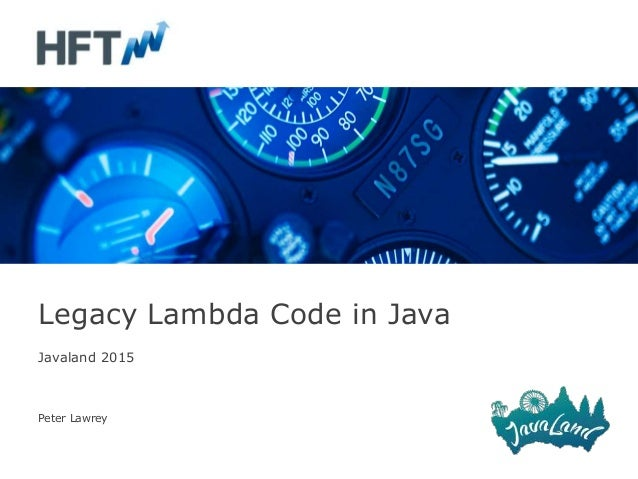Successfully reported this slideshow.
We use your LinkedIn profile and activity data to personalize ads and to show you more relevant ads. You can change your ad preferences anytime.Upcoming SlideShare
×

# Legacy lambda code

1,298 views

Published on

After migrating a three year old C# project to Java we ending up with a significant portion of legacy code using lambdas in Java. What was some of the good use cases, code which could be written better and the problems we had migrating from C#. At the end we look at the performance implications of using Lambdas.

Published in: Technology
• Full Name
Comment goes here.

Are you sure you want to Yes No
Your message goes here• Be the first to comment

### Legacy lambda code

1. 1. Javaland 2015 Peter Lawrey Legacy Lambda Code in Java
2. 2. Agenda • Lambdas: A complicated way to do something simple? • Lambda Patterns we used. • Lambda Patterns we shouldn’t have used. • Lambda Patterns we have used since.
3. 3. Counting elements in a Stream long count = list.stream().count();
4. 4. Counting elements in a Stream long count = list.stream().count(); // Stream.count() @Override public final long count() { return mapToLong(e -> 1L).sum(); }
5. 5. Counting elements in a Stream long count = list.stream().count(); // LongStream.sum() @Override public final long sum() { // use better algorithm to compensate for intermediate overflow? return reduce(0, Long::sum); }
6. 6. Counting elements in a Stream long count = list.parallelStream().count(); When the examples get more complex, lambdas become much more interesting.
7. 7. Porting a legacy C# application For the last 8 months, Higher Frequency Trading ported a legacy C# application with over 25K lines of code to Java. We have translated many LINQ statements into Java 8 Stream + Lambda. What are some common patterns and anti-patterns we have seen?
8. 8. Summing BigDecimal getResults().stream() .reduce(BigDecimal.ZERO, (bd, t) -> bd.add(t.getRequirement()), BigDecimal::add);
9. 9. Validate all entries positions.stream().forEach(Position::validate); Validate throws an exception.
10. 10. Sorting by multiple fields. setTrades(trades.stream() .sorted(comparing(t -> t.getInfo().getDate()) .thenComparing(Position::getCUSIP) .thenComparing(Position::getQuantity) .reversed()) .collect(toList()));
11. 11. Sorting by multiple fields, Quantity reversed setTrades(trades.stream() .sorted(comparing(t -> t.getInfo().getDate()) .thenComparing(Position::getCUSIP) .reversed() .thenComparing(Position::getQuantity) .reversed()) .collect(toList()));
12. 12. Group By Map<String, List<Position>> positionBySymbol = positions.values().stream() .filter(p -> p.getQuantity() != 0) .collect(groupingBy(Position::getSymbol));
13. 13. Streaming Maps pos.entrySet().stream() .filter(p -> p.getValue().getQuantity() != 0.0) .forEach(p -> pos2.put(p.getKey(), p.getValue()));
14. 14. Contains 2.0 if (list.stream() .anyMatch(p -> p.getType() == Type.Cash)) {
15. 15. Deep copy List<Position> newPositions = classPos.stream() .map(Position::clone) .collect(toList())
16. 16. To collect or not to collect (anti-pattern) getTrades().stream() .filter(t -> getDate().equals(t.getInfo().getDate())) .collect(toList()) .forEach(t -> trades.add(t.getInfo()));
17. 17. To collect or not to collect (solution) List<TradeInfo> trades = getTrades().stream() .filter(t -> getDate().equals( t.getInfo().getDate())) .map(Trade::getInfo) .collect(toList());
20. 20. Multi-sorted (anti-pattern) return trade.stream() .filter(t -> !isExcluded(t)) .sorted(comparing(Trade::getDate)) .sorted(comparing(Trade::getCUSIP)) .sorted(comparing(Trade::getNetAmount)) .collect(toList()); See slide 2 example for solution.
21. 21. Concurrent removal ? anti-pattern ? input.stream() .filter(t -> t.getParent() == p.getParent()) .forEach(input::remove);
22. 22. Optional Denial Position todayPos = newPos.stream() .filter(pos -> pos.getCUSIP() .equals(p.getCUSIP())) .findFirst().orElse(null); if (todayPos != null) {